Next: Duality and Qualitative Properties Up: Computational Methods in Finance Previous: Markov Chains

# Expected Values

There are several situations in which expected (present values of) payouts can be computed using an evolution equation that has time moving backwards from the future to the present. The basic idea comes through clearly in the simple case of an undiscounted terminal payout. At the terminal time, T, we get a payout that depends on the state of the system at that time: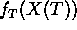. We want to compute the expected value of this payout: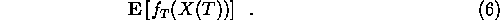To compute this, we compute a connected collection of expectation values, f(x,t), defined as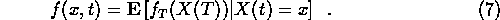We find a relationship between these numbers by considering one step of the Markov chain. If the system is in state x at time t, then the probability for it to be at state y at the next time is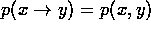. For expectation values, this implies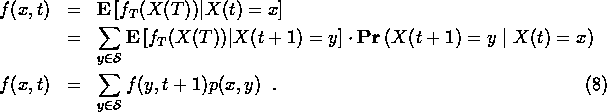This relation is used to compute (5) as follows. The final time values, f(x,T) are the given values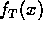. From these, we compute all the numbers f(x,T-1) using (7) with t=T-1. Continuing like this, we eventually get to t=0. We may know X(0), the state of the system at the current time. Otherwise we can use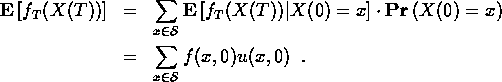All the values on the bottom line should be known.

As with the probability evolution equation (), the equation for the evolution of the expectation values () can be written in matrix form. The difference from the probability evolution equation is that here we arrange the numbers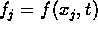into a column vector,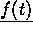. The evolution equation for the expectation values is then written in matrix form as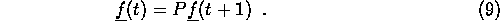This time, the vector goes on the right. If apply () repeatedly, we get, in place of (),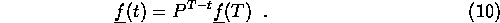There are several useful variations on this theme. For example, suppose that we have a running payout rather than a final time payout. Call this payout g(x,t). If X(t) = x then g(x,t) is added to the total payout that accumulates over time from t=0 to t=T. We want to compute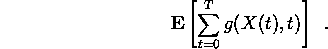As before, we find this by computing more specific expected values: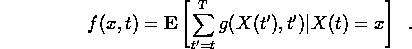These numbers are related through a generalization of (7) that takes into account the known contribution to the sum from the state at time t: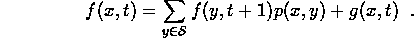The ``initial condition'', given at the final time, is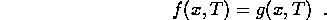This includes the previous case, we take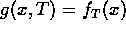and g(x,t) = 0 for t ;SPMlt; T.

As a final example, consider a path dependent discounting. Suppose for a state x at time t there is a discount factor r(x,t) in the range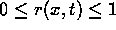. A cash flow worth f at time t+1 will be worth r(x,t)f at time t if X(t) = x. We want the discounted value at time t=0 at state X(0) = x of a final time payout worthat time T. Define f(x,t) to be the value at time t of this payout, given that X(t) = x. If X(t) = x then the time t+1 expected discounted (to time t+1) value is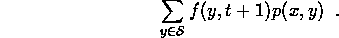This must be discounted to get the time t value, the result being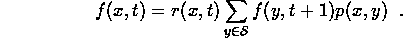Next: Duality and Qualitative Properties Up: Computational Methods in Finance Previous: Markov Chains

Jonathan Goodman
Tue Sep 15 17:12:32 EDT 1998